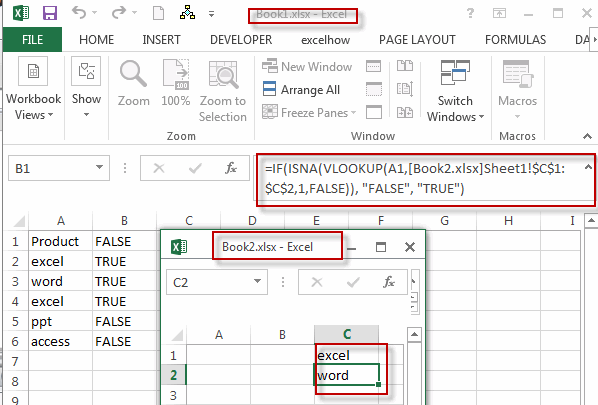# Excel Vlookup From Another Workbook

This post will guide you how to lookup value from another workbook in Excel. How do I use VLOOKUP function across multiple workbooks in Excel. How to vlookup a value from another workbook with a formula in Excel.

## Excel Vlookup From Another Workbook

Before we use the VLOOKUP function to look for a value in current workbook. Assuming that you have request that look for value across multiple workbook. How to achieve it. You can use the VLOOKUP function with a full reference to the other workbook. Like this formula:

`=IF(ISNA(VLOOKUP(A1,[Book2.xlsx]Sheet1!\$C\$1:\$C\$2,1,FALSE)), "FALSE", "TRUE")`

This formula will search the range of cells in Book1.xlsx workbook to look for values in range of cell C1:C2 in workbook Book2.xlsx. if found, return TRUE, otherwise, return FALSE.

The syntax for external references is:

`[Book2.xlsx]Sheet1!\$C\$1:\$C\$2`

The Book2.xlsx is the name of the external workbook.

Sheet1 is the name of the sheet of the external workbook.

!\$C\$1:\$C\$2 is the range of cells that you need to use in the external sheet.

Type this formula in a cell B1 in current worksheet and press Enter key.You will see that the VLOOKUP function will call the data from external workbook(book2.xlsx) and get the expected result in the column B.

### Related Functions

• Excel IF function
The Excel IF function perform a logical test to return one value if the condition is TRUE and return another value if the condition is FALSE. The IF function is a build-in function in Microsoft Excel and it is categorized as a Logical Function.The syntax of the IF function is as below:= IF (condition, [true_value], [false_value])….
• Excel VLOOKUP function
The Excel VLOOKUP function lookup a value in the first column of the table and return the value in the same row based on index_num position.The syntax of the VLOOKUP function is as below:= VLOOKUP (lookup_value, table_array, column_index_num,[range_lookup])….
• Excel ISNA function
The Excel ISNA function used to check if a cell contains the #N/A error, if so, returns TRUE; otherwise, the ISNA function returns FALSE.The syntax of the ISNA function is as below:=ISNA(value)….